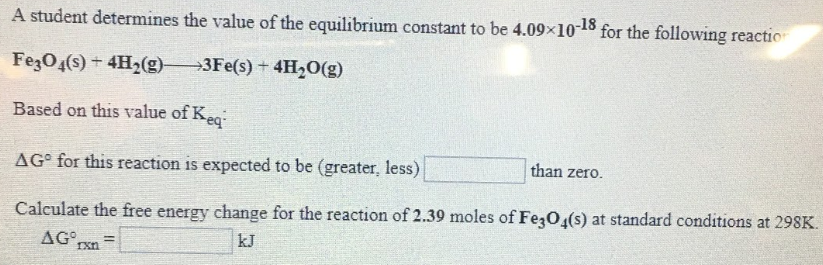# A student determines the value of the equilibrium constant to be 4.09x10^-18 for the following reaction. FeO4(s) +4H2(g) → 3Fe(s) + 4H2O(g) Based on this value of Keq: ΔG° for this reaction is expected to be (greater, less) ____ than zero. Calculate the free energy change for the reaction of 2.39 moles of FeO4(s) at standard conditions at 298K. ΔG°rxn = ____ kJ.